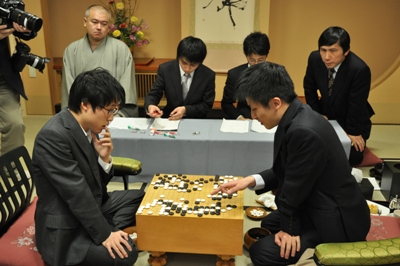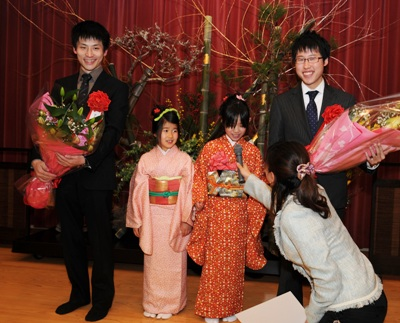## Archive for January, 2011

### Kisei 2011- Cho U Equals the Score in Game 2

Saturday, January 29th, 2011

After losing the first game to challenger Iyama Yuta, Kisei title holder Cho U won the second game.I found Iyama’s move 40 quite amazing, it is played in a place that would never occur to me, see the next diagram.In the game black replied by connecting with the triangle marked stone. This makes move 40 a very efficient forcing move, since now white has even more forcing moves from the outside. This diagram shows the strongest continuation for white – in fact white did play this, but much later in the game.The last diagram shows why black cannot reply aggressively in response to white 40.Here is the complete game.

(; EV[35th Kisei title match 2] KM[6.5] FF SZ GM PW[Iyama Yuta] WR[Meijin] DT[2011-01-26,27] BR[Kisei] RE[B+R] PB[Cho U] ;B[qd];W[dc];B[pq];W[cp];B[nc];W[po];B[qm];W[qq];B[mq];W[pp];B[pr] ;W[ro];B[eq];W[do];B[pj];W[qr];B[ip];W[ci];B[fd];W[hd];B[dd];W[ed] ;B[ee];W[ec];B[cc];W[fe];B[cd];W[ff];B[ef];W[fc];B[cg];W[eg];B[dg] ;W[eh];B[eo];W[en];B[fo];W[dq];B[er];W[be];B[df];W[jp];B[jo];W[kp] ;B[mo];W[io];B[ho];W[in];B[iq];W[kn];B[hn];W[im];B[dn];W[cn];B[dm] ;W[cm];B[dl];W[cl];B[ph];W[ld];B[me];W[qc];B[pc];W[le];B[mf];W[lf] ;B[lg];W[md];B[mh];W[nd];B[od];W[rd];B[rc];W[ob];B[qb];W[ng];B[nh] ;W[kg];B[qn];W[mn];B[qo];W[qp];B[no];W[nn];B[lp];W[ko];B[kr];W[mg] ;B[lh];W[og];B[pg];W[oe];B[oc];W[pf];B[qf];W[qe];B[re];W[pe];B[rg] ;W[kh];B[lb];W[bg];B[bh];W[ah];B[bf];W[af];B[ag];W[rf];B[sf];W[bg] ;B[bi];W[cf];B[ch];W[ce];B[bd];W[de];B[ad];W[ae];B[ai];W[jr];B[jq] ;W[cb];B[ag];W[kq];B[lr];W[ah];B[bb];W[ac];B[bc];W[ba];B[ab];W[oj] ;B[ok];W[db];B[ag];W[pk];B[pl];W[ah];B[dk];W[ca];B[ag];W[nk];B[qk] ;W[ah];B[bo];W[aa];B[co];W[bk];B[ck];W[bj];B[cj];W[di];B[bm];W[bl] ;B[bn];W[dh];B[ag];W[ac];B[bf];W[mb];B[al];W[mc];B[nb];W[na];B[rn] ;W[mj];B[oi];W[gl];B[fk];W[gk];B[on];W[oq];B[or];W[nr];B[nq];W[op] ;B[ns];W[ps];B[mr];W[sp];B[qs];W[rr];B[sn];W[sq];B[fi];W[gh];B[lj] ;W[lk];B[ki])

### Kisei 2011 – Iyama Yuta Wins Game 1

Friday, January 14th, 2011

Iyama Yuta who challenges Cho U for the Japanese Kisei title won game one by resignation.Here is the exchange that took place at the end of the game:The continuation is puzzling though – did Cho U make a mistake, or just set the scene for resignation? Here are the final moves. After black cuts with 6, a and b are miai for black, and he wins the semeai on the upper side.White should have played as in the following diagram. The game looks close to me after this – in fact white looks a little ahead, but maybe I am missing something.Here is the complete game.

(;FFGMSZSTCA[UTF-8]AP[SGFC:1.16] EV[Kisei 2011, game 1] PB[Iyama Yuta] BR[9DP] HA PW[Cho U] WR[9DP] KM[6.5] DT[2011-01-14] RE[B+R] ;B[pd];W[dp];B[qp];W[ed];B[cd];W[cc];B[ic];W[dd];B[ce];W[bc] ;B[cn];W[fp];B[dj];W[oq];B[kq];W[on];B[qm];W[iq];B[ko];W[ol] ;B[pk];W[ok];B[pj];W[oj];B[pi];W[jp];B[kp];W[km];B[jo];W[im] ;B[ho];W[gq];B[hr];W[ir];B[hm];W[in];B[io];W[hn];B[hq];W[gr] ;B[fo];W[hp];B[gn];W[dn];B[dm];W[en];B[il];W[jl];B[jm];W[jn] ;B[kn];W[jm];B[lm];W[ik];B[lk];W[mn];B[ln];W[hl];B[gm];W[fl] ;B[po];W[oo];B[pq];W[pr];B[or];W[nr];B[gp];W[go];B[op];W[nq] ;B[gp];W[hs];B[ep];W[go];B[np];W[mp];B[gp];W[fq];B[eo];W[do] ;B[eq];W[go];B[er];W[dl];B[cq];W[lr];B[kr];W[js];B[mq];W[no] ;B[pp];W[lq];B[lp];W[mo];B[gp];W[cm];B[ls];W[mr];B[go];W[ks] ;B[dg];W[dq];B[dr];W[bq];B[cp];W[co];B[bp];W[bo];B[br];W[gc] ;B[nc];W[ci];B[di];W[cj];B[ch];W[eh];B[ck];W[bk];B[eg];W[fh] ;B[fg];W[gh];B[gg];W[hg];B[hf];W[if];B[he];W[ig];B[ie];W[qd] ;B[qe];W[pc];B[od];W[rc];B[re];W[ob];B[nb];W[qb];B[qr];W[dh] ;B[bh];W[bj];B[hj];W[hi];B[gl];W[ij];B[gk];W[fj];B[rd];W[qc] ;B[hb];W[gb];B[jf];W[jg];B[kf];W[mg];B[kg];W[kh];B[ps];W[pl] ;B[ql];W[pn];B[qn];W[me];B[og];W[ng];B[lh];W[oh];B[ki];W[of] ;B[jh];W[ii];B[pg];W[oi];B[ph];W[ld];B[lc];W[hc];B[ib];W[kc] ;B[kb];W[jd];B[jc];W[kd];B[id];W[mc];B[fk];W[ek];B[lb];W[ae] ;B[fm];W[bf];B[em];W[dk];B[fd];W[fe];B[gd];W[ee];B[ge];W[cf] ;B[eb];W[fc];B[ec];W[db];B[ea];W[ga];B[dc])

### Kisei 2011 Started

Thursday, January 13th, 2011

The most important Japanese title in professional Go, Kisei, started yesterday.

The title holder is Cho U, while the challenger is Iyama Yuta who seems to be in very good shape recently (he defeated Cho U in the final of the Daiwa Cup).Here is the game at the end of day one – Cho U plays white and he made the sealed move. White’s shape in the center looks bad, like damezumari, but he can capture some black stones and fix that.